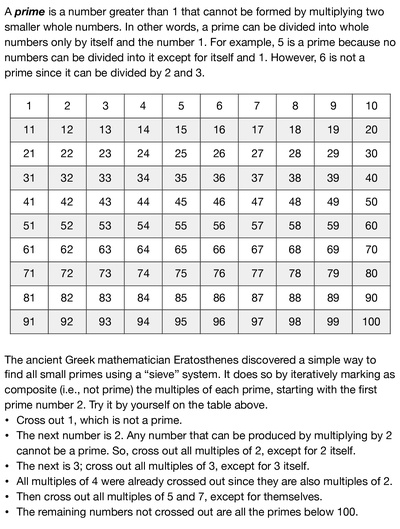# Finding prime numbers by siftingA prime is a number greater than 1 that cannot be formed by multiplying two smaller whole numbers. In other words, a prime can be divided into whole numbers only by itself and the number 1. For example, 5 is a prime because no numbers can be divided into it except for itself and 1. However, 6 is not a prime since it can be divided by 2 and 3. The ancient Greek mathematician Eratosthenes discovered a simple way to find all small primes using a “sieve” system. It does so by iteratively marking as composite (i.e., not prime) the multiples of each prime, starting with the first prime number 2. This worksheet provides a number table from 1 to 100 that kids can use to find primes by applying the sifting algorithm of Eratosthenes.